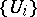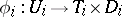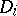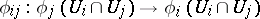Lamination

A topological space partitioned into subsets (called "sheets" ) which look parallel in local charts. More precisely, a lamination is a Hausdorff spacewhich is equipped with a coveringby open subsets and coordinate charts, whereis homeomorphic to a domain in Euclidean space and whereis some topological space. The sheets are the subsets ofwhich are sent locally by the mappingsto the Euclidean factors and the transition mappingsare homeomorphisms which preserve the sheets.

Laminations appear, with slightly distinct meanings and with different uses, in low-dimensional topology (where they have been introduced by W. Thurston) and later on in holomorphic dynamics (where they have been introduced by D. Sullivan).

In low-dimensional topology, laminations are imbedded in manifolds. They generalize the notions of submanifold and of foliation, since a lamination can be seen as a foliation of a (complicated) subset of the manifold. Of special interest are codimension-one laminations (where the sheets are submanifolds of codimension one) on surfaces and on three-dimensional manifolds. The transverse space can be a point, a Cantor set, or a more complicated space.

Codimension-one laminations on surfaces have been especially useful in Thurston's work on the action of the mapping class group on surfaces and on Teichmüller space.

Important classes of laminations include measured laminations and affine laminations, i.e. laminations which carry an invariant transverse measure, respectively an invariant transverse affine structure (see [a3], [a4] and [a6]). In the case of surfaces equipped with hyperbolic structures, another important class of laminations is that of geodesic laminations, i.e. laminations whose sheets are geodesic lines. There are natural correspondences between the theory of measured foliations, that of measured laminations, and that of measured geodesic lamination (in the case of a hyperbolic surface). The space of projective classes of measured laminations is Thurston's boundary of Teichmüller space. F. Bonahon has studied, in a series of papers, laminations which carry transverse Hölder distributions (see, e.g., [a1]).

In three-dimensional manifolds, an important class of codimension-one laminations is the class of essential laminations, which have been introduced by D. Gabai and U. Oertel in [a2], as a generalization of both incompressible surfaces and foliations without Reeb components.

In holomorphic dynamics, Sullivan has introduced the notion of an abstract lamination (that is, there is no ambient manifold for the lamination). In particular, he used the notion of Riemann surface laminations, which plays in conformal dynamics a role similar to that of a Riemann surface in the theory of Kleinian groups (cf. also Kleinian group). A Riemann surface lamination is locally a product of a complex disc times a Cantor set, and Sullivan associates such a lamination to any-smooth expanding mapping of the circle (see [a7]). M. Lyubich and Y. Minsky went one dimension higher, and they introduced hyperbolic three-dimensional laminations in the study of rational mappings of the two-dimensional sphere (see [a5]).